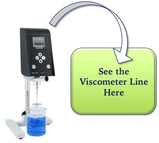# CSC Scientific BlogSubscribe in a reader

# CSC Scientific Blog

At first glance, viscosity seems like a fairly simple concept. It helps describe how thick a product is, or how well it flows. That's all, right?

In reality, there are several different terms that come under the heading of viscosity. These terms are derived from how the viscosity is measured. When people talk about viscosity, they are talking about one of two things: kinematic viscosity or dynamic viscosity.

It's not easy to find a lot of information on the differences between dynamic and kinematic viscosity. This is my attempt to bring clarity to these two principal concepts.

One way is to measure a fluid’s resistance to flow when an external force is applied. This is dynamic viscosity.

The other way is to measure the resistive flow of a fluid under the weight of gravity. The result is kinematic viscosity. Put another way, kinematic viscosity is the measure of a fluid’s inherent resistance to flow when no external force, except gravity, is acting on it.

To further complicate my attempt to simplify these concepts, two fluids that have the same dynamic viscosity can have different kinematic viscosities. This is because kinematic results are dependent on the density of the fluid. Density is not a factor with dynamic viscosity.

## Need a Refresher on Density?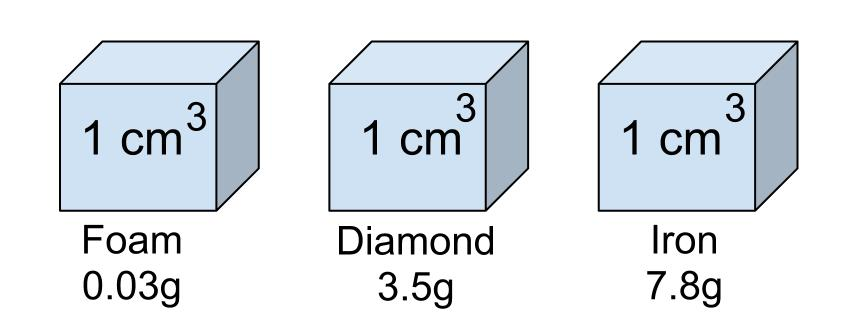Density is the ratio of the mass (or weight) of the sample divided by the volume of the sample. Think about an ice cube and a cube of steel. They may be the same size, but the steel cube weighs more than the ice cube. Therefore, we say that steel has a greater density than ice cube.

The mass (or weight) of a fluid is determined by gravity. In the kinematic measuring method, gravity is the only force that acts on the sample.

## Measuring Dynamic Viscosity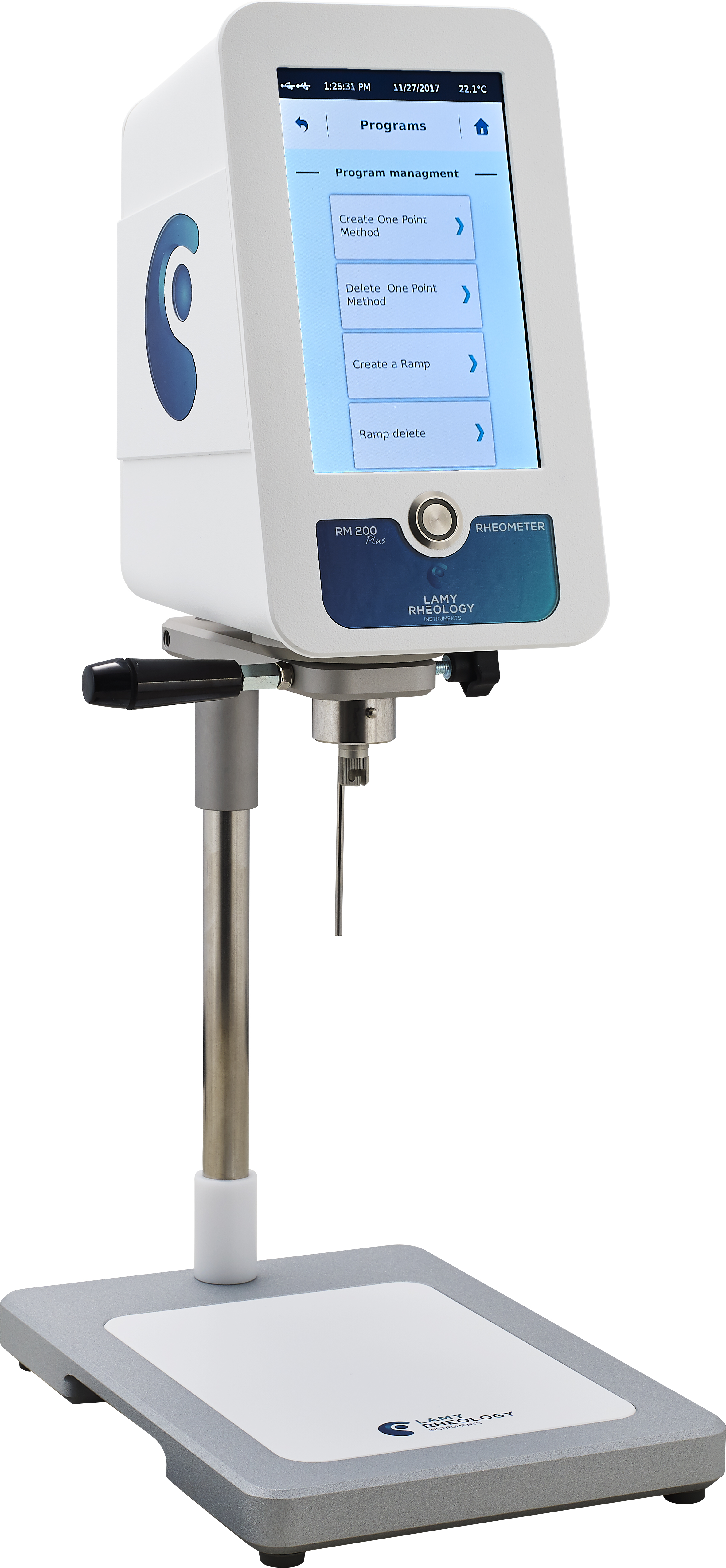Rotational viscometers are one of the more popular types of instruments used to measure dynamic viscosity. These instruments rotate a probe in the liquid sample. Viscosity is determined by measuring the force - or torque - needed to turn the probe.

The rotational viscometer is particularly useful in measuring non-Newtonian liquids. Non-Newtonian liquids change viscosity when exposed to different conditions. For example, some of these liquids show an increase in viscosity with an increase in applied force while other non-Newtonian liquids decrease in viscosity with an increase in applied force.

The rotational viscometer can adjust the turning speed of the probe as it moves in the liquid. The viscometer detects the variation in the viscosity of the sample as the speed, sometimes call shear rate, is changed.

The unit of measure for dynamic viscosity is Centipoise (cP).

## Measuring Kinematic ViscosityThere are several ways to find the kinematic viscosity of a fluid, but the most common method is determining the time it takes a fluid to flow through a capillary tube. The time is converted directly to kinematic viscosity using a calibration constant provided for the specific tube.

The unit of measure of kinematic viscosity is Centistokes (cSt).

A basic difference between the dynamic and kinematic viscosity measurements is density. Density actually provides a way to convert between a kinematic and a dynamic viscosity measurement. The formula for the conversion is:

• Kinematic (cSt) x Density = Dynamic (cP)
• Dynamic (cP) / Density = Kinematic (cSt)

For a given sample, with a density greater than one, dynamic viscosity will always be the higher number.

## When Should You Use Dynamic Viscosity Measurements?

You test dynamic viscosity when you want to know the internal resistance of a fluid, or the force required to move one plane of the liquid over another.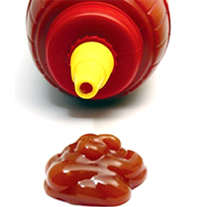The measurement of dynamic viscosity is most useful for liquids which change their apparent characteristics as force or pressure is applied. These liquids are known as non-Newtonian fluids. Non-Newtonian fluids are sensitive to changes in the amount of force exerted on them, and can sometimes even permanently change their viscosity if a constant force has been has been exerted on them over a period of time.

An example of the importance of dynamic viscosity measurement is to indicate the proper flow characteristics of ketchup. This product needs to have lower viscosity as it flows, to get it out of the bottle, but needs to be thick (or not as inclined to flow) when sitting on the burger. Testing the viscosity of the ketchup at different speeds (equating to different levels of force) will help ensure that the ketchup is behaving as it should.

Another application is in the design of pumping systems. Because the viscosity of non-Newtonian liquids changes with the speed of movement, pressure and pump velocity have serious impact on the specification of proper pumps, pressure and piping size. Testing the product at different speeds will help provide guidelines for the design of the pumping system.

## When Should You Use Kinematic Viscosity Measurements?

This measurement is used mostly for Newtonian liquids - liquids that do not change viscosity with changes in applied force (shear rate).

Testing lubricating oils is a significant application. Using this testing method, changes in viscosity at different temperatures and under differing environmental conditions can be determined. With this information, changes in lubricating effectiveness can be evaluated.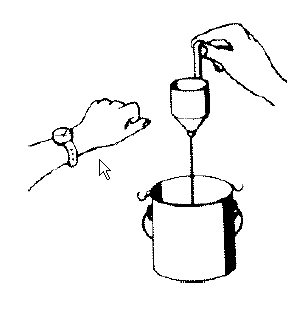Some other products for which the kinematic method is suitable are blood and plasma, paints, polymers, and asphalt.

Viscosity measurement for Newtonian fluids can be accomplished using rotational viscometers (via the conversion formula listed above). However, it is simpler to use capillary-based instruments. In some cases, capillary-based instruments are more accurate for determining kinematic viscosity.

When you need to determine the viscosity characteristics of a liquid which is not exposed to outside physical forces (in other words, when gravity is the only force acting on the liquid), kinematic should be the method of choice.

Summary

Newtonian fluids have an inherent viscosity that does not change as you change the force applied to the liquid. This inherent viscosity can be easily and accurately measured with a capillary-type apparatus, using gravity to move the fluid.

On the other hand, non-Newtonian fluids exhibit wide variations in viscosity based on the force applied. These tests require instruments such as rotational viscometers that can measure changes over time and over a range of applied forces.

To delineate between these two type of liquids:

• Dynamic Viscosity: viscosity related to the external force applied to non-Newtonian fluids.
• Kinematic Viscosity: inherent viscosity of Newtonian fluids, that does not change with a change in applied force.

While this comparison in not exhaustive, I hope it serves to further your understanding of the differences between dynamic viscosity and kinematic viscosity. Please share it with anyone who might be interested.

Until next time,

Amanda

P.S. For more on the difference between Newtonian and non-Newtonian liquids get the PDF.P.P.S.  Be the first to know when we post a new blog article. Subscribe at the top of the page today!